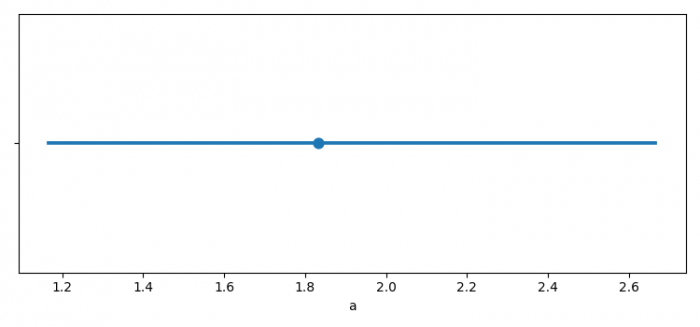# How to get data labels on a Seaborn pointplot?

To get data labels on a Seaborn pointplot, we can take the following steps −

## Steps

• Set the figure size and adjust the padding between and around the subplots.

• Create a dataframe, df, of two-dimensional, size-mutable, potentially heterogeneous tabular data.

• Create a pointplot.

• Get the axes patches and label; annotate with respective labels.

• To display the figure, use show() method.

## Example

from matplotlib import pyplot as plt
import pandas as pd
import seaborn as sns

plt.rcParams["figure.figsize"] = [7.50, 3.50]
plt.rcParams["figure.autolayout"] = True

df = pd.DataFrame({'a': [1, 3, 1, 2, 3, 1]})

ax = sns.pointplot(df["a"],
order=df["a"].value_counts().index)

for p, label in zip(ax.patches, df["a"].value_counts().index):
ax.annotate(label, (p.get_x() + 0.375, p.get_height() + 0.15))

plt.show()

## Output

It will produce the following output −